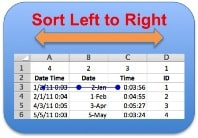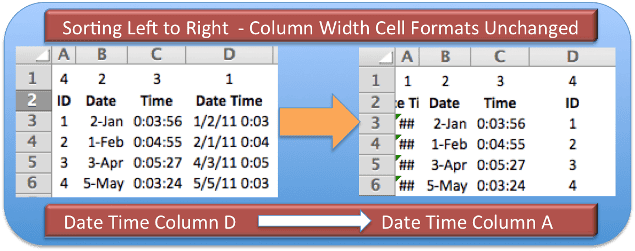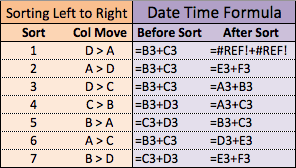# Sorting Columns From Left To Right in Excel Causes ProblemsYou may never have the opportunity to Sort columns of data from Left to Right, but beware of some apparent problems. A reader mentioned that when sorting from left to right the column width’s don’t change. I also found that formulas can be affected and for me that’s more problematic.

I was asked if Excel 2010 had fixed the problem with having column width’s stay the same when Sorting from Left to Right. I didn’t know so I constructed an example to find out. Note: Both Excel 2010 (Windows) and 2011 (Mac) work the same way for the examples that follow.

## Column Width Problem

I used four column headings: ID, Date, Time, and Date Time with some random data, then formatted the cells and adjusted the column widths. I inserted a row above the data and entered the numbers 4, 2, 3, 1 above the headings and Sorted from Left to Right.

In the picture below, the worksheet on the left is Before Sort, and the worksheet on the right is After Sort. The Date Time and ID headings were swapped during the sort. However the column width did not change for column A or D, effectively rendering the Date Time unreadable.## Formula Problems in Sorting Left to Right

In these examples below there’s only one, simple formula. Essentially Date Time equals Date plus Time. (Date Time = Date + Time)

### Formula Problem – Example 1

In this first example cells B3 and C3 have a blue dot and connected lines pointing to cell D3, which contains the formula =B3+C3 and is the active cell. The visual reference is provided by choosing Formulas > Trace Precedents while the active cell contains a formula.

I provided the same Left to Right Sort, swapping columns D and A during the process, and what to my wondering eyes did appear? A broken in formula for Date Time. (And eight tiny reindeer.) 🙂

The formula reference for cell A3 transformed to =#REF!+#REF! after the Sort was completed. Not the kind of thing you want happening.

### Formula Problem – Example 2

In this second example I’ve corrected the formula in cell A3, which is now =B3+C3. This time I’ll Sort from Left to Right using Row 1 to sort from Smallest to Largest. The result will be columns A and D trading places. (Can I get a movie reference here? Don’t worry, I’m almost done.)

The spreadsheet below depicts what happened to the formula in cell D3, which is now =E3+F3 and obviously wrong again.

### Formula Problem – Test Summary

With four columns, swapping one formula back and forth to see what happens could take some time. I compiled a small, sample summary table with 7 different sorts showing the Date Time formula before and after sorting the columns from left to right.Col Move shows how the formula cell changed columns. In each case, after sorting the formula was incorrect. Some of the time there was one cell, either Date or Time, that was correct in the formula, but I didn’t find a combination that left the formula fully functional. I didn’t do an exhaustive combination so there may be some sorts that don’t break the formula, but I’ve made my point here.

Be very careful of formulas when sorting columns from left to right. And, oh yeah, you’ll probably be auto-fitting column widths as well.